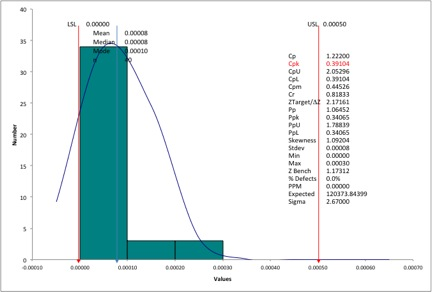# Calculating Cpk for Runout (and other GD&T specifications)

A few days ago we received an email from a friend at a machine shop. He had just finished a process capability (Cp, Cpk) analysis for a critical feature (a runout on a cylindrical part) and was shocked by the output. The very popular spreadsheet software he used showed him a Cpk of 0.39.Runout Data with Cpk = 0.39 (incorrect)

To put this in perspective, with a Cpk of 0.39, he should be seeing a defect rate of between 12% and 24% (assuming normality of data). However, he was actually experiencing a defect rate close to zero. In fact, he hadn’t had any problems with runout as far as he could remember.

So why was the software telling him that his Cpk was 0.39? And what was his real Cpk?

### Sources of errors

In general, we find two sources of error during process capability analysis:

1. The practitioner has violated one of the assumptions required for proper analysis (you can see a list of assumptions here: "pitfalls to avoid with process capability analysis")
2. The practitioner has not taken into consideration the "nature of the characteristic" being measured, and has used an incorrect formula.

### The nature of the characteristic

Runouts are specified with a single value: a not-to-exceed value. For example, the specification for runout could be 0.0005 inches. This means that the Upper Spec Limit (or USL) = 0.0005 in. But what is the Lower Spec Limit? And what is the Nominal?

The Lower Spec Limit is obviously zero. In fact zero is a hard limit; you cannot have a runout value less than zero. In addition, the Nominal (or the target value) is also zero. In other words, in the ideal case, we should not have any runout.

Notice that the Nominal is NOT mid-way between the USL and the LSL. Instead the Nominal = LSL. These two facts, a hard LSL of zero, and a Nom = LSL, make runout a very special feature.

To make matters worse, runout data is often not normally distributed. Instead of a symmetrical distribution with a hump in the middle, we see a skewed distribution. A typical runout distribution will have a large number of values at or close to zero (the LSL) and fewer values closer to the USL.

### Two challenges with runouts

So now, we have identified two challenges with calculating Cpk for runouts:

1. The Nom = LSL = zero
2. A non-normal distribution

Let’s deal with the first problem. Our goal is to have the process mean located on the Nominal. In other words, the mean should be closer to the LSL and far from the USL. So our Cpk needs to be calculated as the distance from the USL to the process mean.

The metric we use is the Cpu, and assuming that we have a normal distribution, we calculate:

Cpk = Cpu = (USL – Mean)/(3 * SigmaRSG),

where, SigmaRSG is the rational subgroup estimate of sigma (often referred to as the short-term standard deviation).

We will address the second challenge (non-normal data) in a later post. For now, we’ll operate under the assumption that the process is normally distributed.

### Dramatically different results

When we recalculated the Cpk for our friend using the formula above, we found that the Cpk was actually 2.05. That is a six-sigma defect level, and explains why our friend was seeing no defects.

So remember, before you begin calculating Cpk, check your assumptions, and think about the nature of the characteristic being measured.